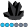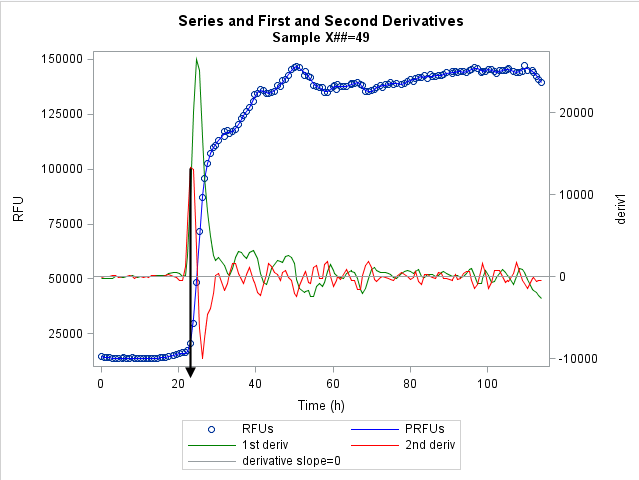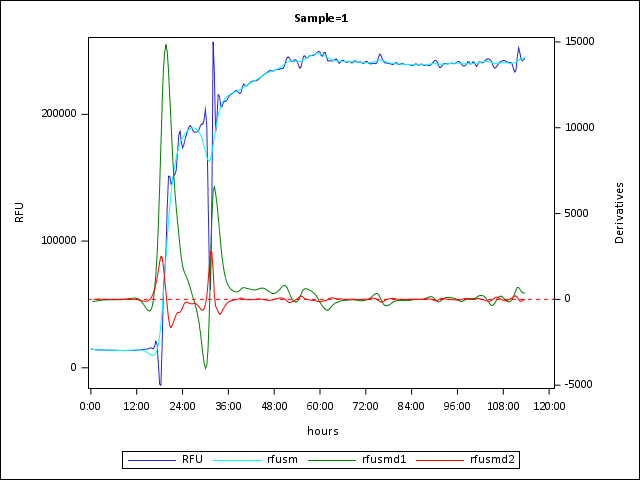das
Obsidian | Level 7

## Procedure to derive inflection points from Loess fit of data?

Can I use a SAS procedure(s) to determine the (x,y) coordinates of a non-linear complex curve (no model, just LOESS fit)?

The raw data: Process monitored as Relative Fluorescence Units (RFUs) captured over time.

Main goal: Use SAS to determine the lag phase of the process (time to first major inflection point).

What I have achieved so far (see plot below): Import the raw data (blue circles) and reduce its noise using PROC LOESS to output predicted values (PRFUs; blue line). Derive the first derivative (green line) and second derivative (red line) of the PRFU data.

As indicated by black arrow in the plot, the time position of the first major positive maximum of the second derivative would be a nice objective way to estimate the lag phase. Can I accomplish this in SAS? Is there a better approach (in SAS)?

I think I am looking for a procedure to which i can pass the second derivative of the data and have it return the (x,y) coordinates of maxima.

Thank you,

Dave1 ACCEPTED SOLUTION

Accepted Solutions

## Re: Procedure to derive inflection points from Loess fit of data?

First, read the article about how to compute derivatives for nonparametric regression models. That provides the basics of finite difference derivatives. To tell the difference between extrema and inflection points, you need to compute the numerical second derivatives. Warren's article on processing ODS output data set gives an example, although his article is mostly concerned with using ODS OUTPUT to capture the data from a plot. Presumably, you can get the curve directly, and don't need to use ODS OUTPUT to get the curve values.

9 REPLIES 9

## Re: Procedure to derive inflection points from Loess fit of data?

If the SIGN (+ or -) of y2-y1 is different than that of y3-y2 then you have crossed a local maxima or minima

So something like

data want;

set have;

d1 = lag1(y) - lag2(y);

d   = y - lag1(y);

if sign(d1) ne sign(d) then <whatever you want to do with candidate points>.

run;

may get you started.

But that is going to require actual data points so you would need smoothed data.PGStats
Opal | Level 21

## Re: Procedure to derive inflection points from Loess fit of data?

PGdas
Obsidian | Level 7

## Re: Procedure to derive inflection points from Loess fit of data?

Yes, I was able to run Example 8.4 "missing values" out of the documentation for the AUTOREG Procedure. I am running SAS9.3 TS Level 1M2.

I've attached a small portion of an experiment (data are for three independent samples; i.e., these are not replicates but different concentrations of a starting seed for the reaction).

BTW, i am also starting to look at modeling with something like Gompertz, but i'd rather not make the model assumptions at this point. Thus applying smoothing rather than modeling.

Thank you,

DavePGStats
Opal | Level 21

## Re: Procedure to derive inflection points from Loess fit of data?

I hope this works with your version. Here is a relatively simple way to find the inflexion points using proc expand

``````data rfu;
infile "&sasforum\datasets\rfu.csv" dsd firstobs=2;
input hours RFU Sample;
hours = hours * '01:00:00't; /* Convert to proper SAS time value */
format hours hhmm7.;
run;

/* Regularize to every 20 mins, smoothe, and derive twice */
proc expand data=rfu out=rfuts to=minute20 ;
by sample;
id hours;
convert rfu / observed=(beginning,beginning);
/* Smoothed rfu */
convert rfu=rfusm / transformout=(HP_T 100);
/* First derivative of the smoothed rfu */
convert rfu=rfusmd1 / transformout=(HP_T 100 DIF);
/* Second derivative of the smoothed rfu */
convert rfu=rfusmd2 / transformout=(HP_T 100 DIF DIF);
run;

proc sgplot data=rfuts;
by sample;
series x=hours y=rfu;
series x=hours y=rfusm / lineattrs=(pattern=solid color=cyan);
series x=hours y=rfusmd1 / y2axis lineattrs=(pattern=solid color=green);
series x=hours y=rfusmd2 / y2axis lineattrs=(pattern=solid color=red);
xaxis type=time valuesformat=hhmm7.;
y2axis label="Derivatives";
refline 0 / axis=y2 lineattrs=(pattern=shortdash color=red);
run;

proc sql;
/* Get the top 10 percent of the first derivarives */
create table peaks as
select  Sample, hours format=hhmm7., RFU, rfusmd1, rfusmd2
from rfuts
group by sample
having rfusmd1 > 0.9 * max(rfusmd1)
order by sample, hours;
/* Get the time when the second derivative crosses zero */
title "Time of major inflexion points";
select * from peaks
group by sample
having abs(rfusmd2) = min(abs(rfusmd2));
run;
``````PGdas
Obsidian | Level 7

## Re: Procedure to derive inflection points from Loess fit of data?

Yes, this works well. I like also the suggestion from Rick and some other info I came across, using the PROC MEANS to give me the max values for the first and second derivatives, including the time and predicted RFU's at those inflections.

Thank you very much for your help.

Dave

## Re: Procedure to derive inflection points from Loess fit of data?

First, read the article about how to compute derivatives for nonparametric regression models. That provides the basics of finite difference derivatives. To tell the difference between extrema and inflection points, you need to compute the numerical second derivatives. Warren's article on processing ODS output data set gives an example, although his article is mostly concerned with using ODS OUTPUT to capture the data from a plot. Presumably, you can get the curve directly, and don't need to use ODS OUTPUT to get the curve values.das
Obsidian | Level 7

## Re: Procedure to derive inflection points from Loess fit of data?

I've managed to use the sgplot pbspline maxpoints method to the desired effect if fitting a single sample. But the lag function is populated when it reruns the derivative equations on the next sample (using a 'by sample;'). I see in the help manual that you can set the first values of der1 and der2 to missing, but this does not correct for the second calculation of der2 because of the use of the lag2 option. Is there a simple way to empty the lag queue rather than set calculated values to missing at first instance?

For the moment, i just added a conditional output at the end of the data step so that only values associated with a time greater than the second instance are saved.

Dave

## Re: Procedure to derive inflection points from Loess fit of data?

It sounds like you might be able to use the FIRST.variable and LAST.variable automatic variables to perform special handling for the first element of each BY group.das
Obsidian | Level 7

## Re: Procedure to derive inflection points from Loess fit of data?

Rick,

Thank you for the suggestion and link. I really should search the blog posts as much as the user manual.

Turns out I was making two mistakes. First, was re-setting the wrong variables to missing (was resetting der1 and der2 instead of pbx and pby). Probably more important was the fact that i was placing the resetting lines of code after the calculations of der1 and der2. Here is the code that is now producing the 'inf' data set as I was hoping when using a by statement:

``````data inf ;
set sg(rename=(&ren));
by sample ;
if last.sample then do;
pby=.;
pbx=.;
end;
der1 = (pby - lag(pby)) / (pbx - lag(pbx));
der2 = (lag2(pby) - 2*lag(pby) + pby) / (pbx - lag(pbx)) ** 2;
run;``````

Thank you and thanks to the community for all the help. I keep learning new things AND getting my work done.

Dave

Discussion stats
• 9 replies
• 2421 views
• 1 like
• 4 in conversation